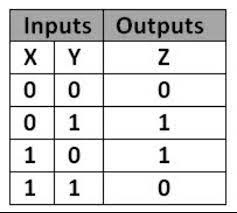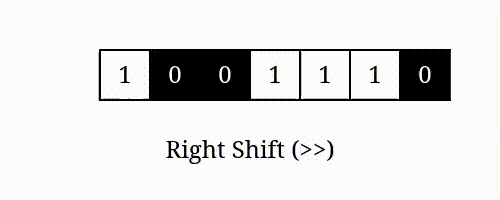# Hamming Distance

## Introduction

Before we proceed any further, we must know what the hamming distance is. The Hamming Distance between two integers is the number of bits in both numbers that differ at the same position.

Before proceeding further, if you want to take a look at how bit operators work, you can have a look at the blog on bit manipulation.

In the following section, let’s dig deeper into the problem statement.

## Problem Statement

We are given two integers. We need to find the hamming distance between these two integers.

### Example:

Suppose two given integers are ‘x’=1 and ‘y’=4.

The hamming distance between two numbers ‘1’ and ‘4’ is ‘2’.

### Explanation:

The binary representation of 1 is  (0 0 0 1)

The binary representation of 4 is  (0 1 0 0)

↑     ↑

Here, only bits at positions ‘0’ and ‘2’ ( zero-based indexing ) are different, so the Hamming Distance is ‘2’.

## Approach: Using XOR

Since we want to compare the bits in the binary representation of two numbers, the most apparent approach is using xor of the numbers.

We know that ‘xor’ has the following properties:Source: brainly

We can observe that whenever the two bits are different, xor gives us 1. That is our intuition for using xor in the given problem.

Algorithm:

1. Initialize a variable ‘hammingCount’ as 0.
2. Take xor of two numbers x and y and store them in a variable called ‘num.’
3. XOR Between the Numbers would give ‘1’ where bits are different; therefore, counting set bits in ‘num’ would provide us with the hamming distance.
4. Now, to count the number of Set bits in ‘num,’ initialize a while loop with the condition while (num>0).
5. At each iteration of the while loop, calculate ‘num & 1’. If it evaluates to ‘1’, then increment ‘hammingCount.’
6. After each iteration, right shift ‘num’ by 1.

For example- The number 78 whose binary representation is 1001110.
When 78 is right shifted by 1 i.e. (78>>1),

it becomes 78/2 = 39 (100111).7. Finally, return ‘hammingCount.’

Wondering how bit manipulation and shift operators work?

Watch one of the best tutorials on Bit Manipulation and Shift Operators by Parikh Jain, co-founder of Coding Ninjas.

Output

### Complexity Analysis

#### Time Complexity:O(N)

Where N is the number of bits in the binary representation of the number.

XOR between 2 numbers is an O(1) Operation.

Since we are iterating over the total number of bits of a number that is 32 bits. The while loop runs for a total of 32 iterations, and for each iteration, we did some constant work which is O(1),

#### Space Complexity:O(1)

We didn’t require any additional space as such. We just created two integer variables which are a constant O(1) space.

1. What if the number cannot be represented in 32 bits means the number is very large?

In that case, we should initialize an array of larger sizes. The Largest integer that we can store in C and C++ is of size 64 bits. So an array of size 64 would help us achieve our target when we encounter large numbers.

.2. What is the time complexity of doing XOR of two numbers?

XOR of two numbers is a constant O(1) operation.

## Key Takeaways

Here we learned one of the most important concepts of bit manipulation in programming called Xor. Xor has many applications in questions involving bitmasking.

Hamming distance between two integers is just the number of positions at which the bits are different in the binary representation of the numbers.

Want to ace the coding rounds of big tech companies? Try our Attempt Unlimited Online Mock Test Series to start your preparation.